How Cheenta works to ensure student success?
Explore the Back-Story

# Limit of a function | IIT JAM 2017 | Problem 8Try this problem from IIT JAM 2017 exam. It deals with evaluating Limit of a function.

## Limit of a Function | IIT JAM 2017 | Problem 8

Let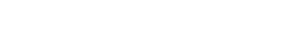Write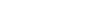and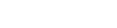Then which one of the following is true?

•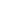exists but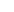does not exist
•does not exist butexists
• Bothandexist
• Neithernorexists

### Key Concepts

Real Analysis

Function

Limit

Answer:exists butdoes not exists

IIT JAM 2017 , Problem 8

## Try with Hints

Given that,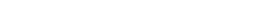therefore,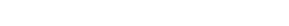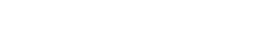Let,and ,Now,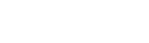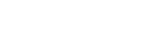Theorem : If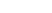and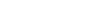. Let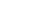. If f is bounded on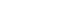and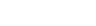, then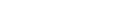.

Now ,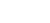is bounded in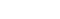and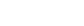, then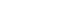exists and equal to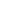.

But,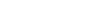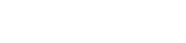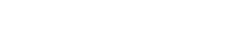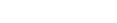does not exists [Why?]

Thenexists butdoes not.

## Subscribe to Cheenta at Youtube

Try this problem from IIT JAM 2017 exam. It deals with evaluating Limit of a function.

## Limit of a Function | IIT JAM 2017 | Problem 8

LetWriteandThen which one of the following is true?

•exists butdoes not exist
•does not exist butexists
• Bothandexist
• Neithernorexists

### Key Concepts

Real Analysis

Function

Limit

Answer:exists butdoes not exists

IIT JAM 2017 , Problem 8

## Try with Hints

Given that,therefore,Let,and ,Now,Theorem : Ifand. Let. If f is bounded onand, then.

Now ,is bounded inand, thenexists and equal to.

But,does not exists [Why?]

Thenexists butdoes not.

## Subscribe to Cheenta at Youtube

This site uses Akismet to reduce spam. Learn how your comment data is processed.

### Knowledge Partner